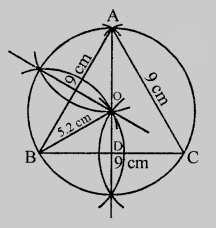# An equilateral triangle of side $9\ cm$ is inscribed in a circle. Find the radius of the circle.

Given:

An equilateral triangle of side $9\ cm$ is inscribed in a circle.

To do:

We have to find the radius of the circle.

Solution:Steps of construction:

1. Draw a line segment $BC = 9\ cm$.

2. With centres $B$ and $C$, draw arcs of $9\ cm$ radius which intersect each other at $A$.

3. Join $AB$ and $AC$.

$\triangle ABC$ is the required triangle.

4. Draw perpendicular bisectors of sides $AB$ and $BC$ which intersect each other at $O$.

5. With centre $O$ and radius $OB$, draw a circle which passes through $A, B$ and $C$.

This is the require circle in which $\triangle ABC$ is inscribed.

On measuring the radius is $5.2\ cm$.

Radius $=\frac{2}{3} \mathrm{AD}$

$=\frac{2}{3} \times \frac{\sqrt{3}}{2} \times \operatorname{side}$

$=\frac{2}{3} \times \frac{\sqrt{3}}{2} \times 9$

$=3 \sqrt{3} \mathrm{~cm}$

$=3 \times 1.732$

$=5.196$

$=5.2 \mathrm{~cm}$

The radius of the circle is $5.2\ cm$.

Updated on: 10-Oct-2022

55 Views